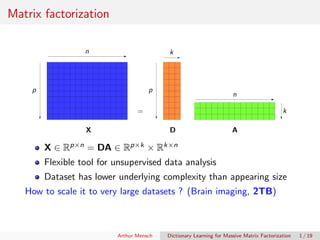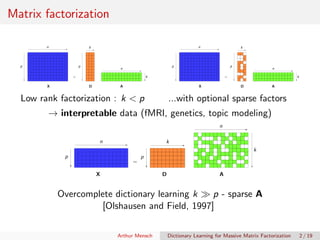Successfully reported this slideshow.

# Dictionary Learning for Massive Matrix Factorization

1

Share×
1 of 25
1 of 25

# Dictionary Learning for Massive Matrix Factorization

1

Share

New methods to perform matrix factorization on large and high-dimensional datasets

New methods to perform matrix factorization on large and high-dimensional datasets

## More Related Content

### Related Books

Free with a 14 day trial from Scribd

See all

### Related Audiobooks

Free with a 14 day trial from Scribd

See all

### Dictionary Learning for Massive Matrix Factorization

1. 1. Dictionary Learning for Massive Matrix Factorization Arthur Mensch, Julien Mairal, Ga¨el Varoquaux, Bertrand Thirion Inria/CEA Parietal, Inria Thoth June 20, 2016
2. 2. Matrix factorization X p n D p k = A n k 1X ∈ Rp×n = DA ∈ Rp×k × Rk×n Flexible tool for unsupervised data analysis Dataset has lower underlying complexity than appearing size How to scale it to very large datasets ? (Brain imaging, 2TB) Arthur Mensch Dictionary Learning for Massive Matrix Factorization 1 / 19
3. 3. Matrix factorization X p n D p k = A n k 1 Low rank factorization : k < p X p n D p k = A n k 1 ...with optional sparse factors → interpretable data (fMRI, genetics, topic modeling) X p n D p k = A n k 1Overcomplete dictionary learning k p - sparse A [Olshausen and Field, 1997] Arthur Mensch Dictionary Learning for Massive Matrix Factorization 2 / 19
4. 4. Formalism and methods Non-convex formulation min D∈C,A∈Rk×n X − DA 2 2 + λΩ(A) Constraints on D Penalty on A ( 1, 2) Naive resolution Alternated minimization: use full X at each iteration Very slow : single iteration in O(p n) Arthur Mensch Dictionary Learning for Massive Matrix Factorization 3 / 19
5. 5. Online matrix factorization Stream (xt), update D at each t [Mairal et al., 2010] Single iteration in O(p), a few epochs xt p n D p k = αt n k streaming 1 Large n, regular p, eg image patches: p = 256 n ≈ 106 1GB Both (sparse) low-rank factorization / sparse coding Arthur Mensch Dictionary Learning for Massive Matrix Factorization 4 / 19
6. 6. Scaling-up for massive matrices Functional MRI (HCP dataset) Brain “movies” : space × time Extract k sparse networks p = 2 · 105 n = 2 · 106 2 TB Way larger than vision problems Unusual setting: data is large in both directions Also useful in collaborative ﬁltering X Voxels Time = D A k spatial maps Time x Arthur Mensch Dictionary Learning for Massive Matrix Factorization 5 / 19
7. 7. Scaling-up for massive matrices Out-of-the-box online algorithm ? xt p n D p k = αt n k 1Limited time budget ? Need to accomodate large p 235 h run time 1 full epoch 10 h run time 1 24 epoch Arthur Mensch Dictionary Learning for Massive Matrix Factorization 6 / 19
8. 8. Scaling-up in both directions X p n Batch → online xt n Steaming Handle large n 1 xt p n Streaming Mtxt n Streaming Subsampling Handle large p Online → double online 1 Online learning + partial random access to samples Arthur Mensch Dictionary Learning for Massive Matrix Factorization 7 / 19
9. 9. Scaling-up in both directions xt p n Streaming Mtxt n Streaming Subsampling Handle large p Online → double online 1 Low-distorsion lemma [Johnson and Lindenstrauss, 1984] Random linear alebra [Halko et al., 2009] Sketching for data reduction [Pilanci and Wainwright, 2014] Arthur Mensch Dictionary Learning for Massive Matrix Factorization 8 / 19
10. 10. Algorithm design Online dictionary learning [Mairal et al., 2010] 1 Compute code – O(p) αt = argmin α∈Rk xt − Dt−1α 2 2 + λΩ(αt) 2 Update surrogate – O(p) gt = 1 t t i=1 xi − Dαi 2 2 3 Minimize surrogate – O(p) Dt = argmin D∈C gt(D) = argmin D∈C Tr (D DAt − D Bt) xt access → O(p) algorithm (complexity dependency in p) Arthur Mensch Dictionary Learning for Massive Matrix Factorization 9 / 19
11. 11. Introducing subsampling Iteration cost in O(p): can we reduce it? xt → Mtxt, p → rk Mt = s Use only Mtxt in algorithm computation: complexity in O(s) Mtxt p n Streaming Subsampling 1Our contribution Adapt the 3 parts of the algorith to obtain O(s) complexity 1 Code computation 2 Surrogate update 3 Surrogate minimization [Szab´o et al., 2011]: dictionary learning with missing value – O(p) Arthur Mensch Dictionary Learning for Massive Matrix Factorization 10 / 19
12. 12. 1. Code computation Linear regression with random sampling αt = argmin α∈Rk Mt(xt − Dt−1αt) 2 2 + λ rk Mt p Ω(α) approximative solution of αt = argmin α∈Rk xt − Dt−1αt 2 2 + λΩ(α) validity in high dimension, with incoherent features: D MtD ≈ s p D D D Mtxt ≈ s p D xt Arthur Mensch Dictionary Learning for Massive Matrix Factorization 11 / 19
13. 13. 2. Surrogate update Original algorithm: At and Bt used in dictionary update At = 1 t t i=1 αi αi same as in online algorithm Bt = (1 − 1 t )Bt−1 + 1 t xtαt = 1 t t i=1 xi αi Forbidden Partial update of Bt at each iteration Bt = 1 t i=1 Mi t i=1 Mi xi αi Only MtB is updated Behaves like Ex[xα] for large t Arthur Mensch Dictionary Learning for Massive Matrix Factorization 12 / 19
14. 14. 3. Surrogate minimization Original algorithm : block coordinate descent with projection on C min D∈C gt(D) Dj ← p⊥ Cj (Dj − 1 Aj,j (D(At)j − (Bt)j )) Forbidden update of full D at iteration t Cautious update Leave dictionary unchanged for unseen features (I − Mt) min D∈C (I−Mt )D=(I−Mt )Dt−1 gt(D) O(s) update in block coordinate descent Dj ← p⊥ Cr j (Dj − 1 (At)j,j (Mt(D(At)j − (Bt)j ))) 1 ball Cr j = {D ∈ C, MtD 1 ≤ MtDt−1 1} Arthur Mensch Dictionary Learning for Massive Matrix Factorization 13 / 19
15. 15. Resting-state fMRI HCP dataset One brain image per second 200 sessions n = 2 · 106 p = 2 · 105 D AX Voxels Time = k spatial maps Time x Sparse decomposition k = 70 C = Bk 1 Ω = · 2 2 Validation Increase reduction factor p s Objective function on test set vs CPU time Arthur Mensch Dictionary Learning for Massive Matrix Factorization 14 / 19
16. 16. Resting-state fMRI Online dictionary learning 235 h run time 1 full epoch 10 h run time 1 24 epoch Proposed method 10 h run time 1 2 epoch, reduction r=12 Qualitatively, usable maps are obtained 10× faster Arthur Mensch Dictionary Learning for Massive Matrix Factorization 15 / 19
17. 17. Resting-state fMRI .1 h 1 h 10 h 100 h CPU time 2.20 2.25 2.30 2.35 2.40 Objectivevalueontestset ×108 Original online algorithm Reduction factor r 4 8 12 No reduction Speed-up close to reduction factor p s Arthur Mensch Dictionary Learning for Massive Matrix Factorization 16 / 19
18. 18. Resting-state fMRI 100 1000 Epoch 4000Records 2.16 2.17 2.18 2.19 2.20 2.21 2.22 2.23 2.24 Objectivevalueontestset ×108 λ = 10−4 No reduction (original alg.) r = 4 r = 8 r = 12 Convergence speed / number of seen records Information is acquired faster Arthur Mensch Dictionary Learning for Massive Matrix Factorization 17 / 19
19. 19. Collaborative ﬁltering Mtxt movie ratings from user t vs. coordinate descent for MMMF loss (no hyperparameters) 100 s 1000 s 0.93 0.94 0.95 0.96 0.97 0.98 0.99 Netﬂix (140M) Coordinate descent Proposed (full projection) Proposed (partial projection) Dataset Test RMSE Speed CD MODL -up ML 1M 0.872 0.866 ×0.75 ML 10M 0.802 0.799 ×3.7 NF (140M) 0.938 0.934 ×6.8 Outperform coordinate descent beyond 10M ratings Same prediction performance Speed-up 6.8× on Netﬂix Arthur Mensch Dictionary Learning for Massive Matrix Factorization 18 / 19
20. 20. Conclusion Take-home message Loading stochastic subsets of sample streams can drastically accelerates online matrix factorization Mtxt p n Streaming Subsampling 1 Reduce CPU (+IO) load at each iteration cf Gradient Descent vs SGD An order of magnitude speed-up on two diﬀerent problems Python package http://github.com/arthurmensch/modl Heuristic at contribution time A follow-up algorithm has convergence guarantees Questions ? (Poster # 41 this afternoon) Arthur Mensch Dictionary Learning for Massive Matrix Factorization 19 / 19
21. 21. Bibliography I [Halko et al., 2009] Halko, N., Martinsson, P.-G., and Tropp, J. A. (2009). Finding structure with randomness: Probabilistic algorithms for constructing approximate matrix decompositions. arXiv:0909.4061 [math]. [Johnson and Lindenstrauss, 1984] Johnson, W. B. and Lindenstrauss, J. (1984). Extensions of Lipschitz mappings into a Hilbert space. Contemporary mathematics, 26(189-206):1. [Mairal et al., 2010] Mairal, J., Bach, F., Ponce, J., and Sapiro, G. (2010). Online learning for matrix factorization and sparse coding. The Journal of Machine Learning Research, 11:19–60. [Olshausen and Field, 1997] Olshausen, B. A. and Field, D. J. (1997). Sparse coding with an overcomplete basis set: A strategy employed by V1? Vision Research, 37(23):3311–3325. Arthur Mensch Dictionary Learning for Massive Matrix Factorization 20 / 19
22. 22. Bibliography II [Pilanci and Wainwright, 2014] Pilanci, M. and Wainwright, M. J. (2014). Iterative Hessian sketch: Fast and accurate solution approximation for constrained least-squares. arXiv:1411.0347 [cs, math, stat]. [Szab´o et al., 2011] Szab´o, Z., P´oczos, B., and Lorincz, A. (2011). Online group-structured dictionary learning. In Proceedings of the IEEE Conference on Computer Vision and Pattern Recognition, pages 2865–2872. IEEE. [Yu et al., 2012] Yu, H.-F., Hsieh, C.-J., and Dhillon, I. (2012). Scalable coordinate descent approaches to parallel matrix factorization for recommender systems. In Proceedings of the International Conference on Data Mining, pages 765–774. IEEE. Arthur Mensch Dictionary Learning for Massive Matrix Factorization 21 / 19
23. 23. Appendix
24. 24. Collaborative ﬁltering Streaming uncomplete data Mt is imposed by user t Data stream : Mtxt movies ranked by user t Proposed by [Szab´o et al., 2011]), with O(p) complexity Validation: Test RMSE (rating prediction) vs CPU time Baseline: Coordinate descent solver [Yu et al., 2012] solving related loss n i=1 ( Mt(Xt − Dαt) 2 2 + λ αt 2 2) + λ D 2 2 Fastest solver available apart from SGD – no hyperparameters Our method is not sensitive to hyperparameters Arthur Mensch Dictionary Learning for Massive Matrix Factorization 22 / 19
25. 25. Algorithm Our algorithm 1 Code computation αt = argmin α∈Rk Mt (xt − Dt−1α) 2 2 + λ rk Mt p Ω(αt ) 2 Surrogate aggregation At = 1 t t i=1 αi αi Bt = Bt−1 + 1 t i=1 Mi (Mt xt αt − Mt Bt−1) 3 Surrogate minimization Mt Dj ← p⊥ Cj (Mt Dj − 1 (At )j,j Mt (D(At )j −(Bt )j )) Original online MF 1 Code computation αt = argmin α∈Rk xt − Dt−1α 2 2 + λΩ(αt ) 2 Surrogate aggregation At = 1 t t i=1 αi αi Bt = Bt−1 + 1 t (xt αt − Bt−1) 3 Surrogate minimization Dj ← p⊥ Cr j (Dj − 1 (At )j,j (D(At )j −(Bt )j )) Arthur Mensch Dictionary Learning for Massive Matrix Factorization 23 / 19